# Selecting the proper diodes for an antilog circuit

#### sindre jacobsen

Joined Apr 1, 2019
40
Hello,
I am trying to make an antilog detector that is as accurate as possible at certain points, (Vshape, Vantilog). A couple of these points that should be upheld are:
@ Vshape = 0.95V, Vantilog ~ 2.0V
@ Vshape = 0.779V, Vantilog ~ 0.632V
By simulating with ideal diodes and ADA4807, these values are closely met (assuming the ideality factor is 1 and the thermal voltage is 26mV). When I try using the diodes 1N4148, the slope of the function has been reduced. I know the ideality factor has increased (1.752 in ltspice), which I've taken into consideration and adjusted the resistances that decides the exponential value accordingly. The function is still quite slack compared to "ideal", and I was wondering if any of you know of any diodes better suited for the application, or a solution that could make the 1N4148 diodes get a more ideal functionality.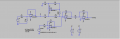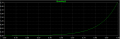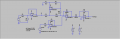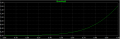S.

#### MrChips

Joined Oct 2, 2009
23,228
You can fit an infinite number of lines to two data points.
You need to provide more data points, minimum of five points.

#### sindre jacobsen

Joined Apr 1, 2019
40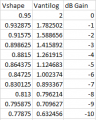The points are given in 1dB decrement (dB reduction from max value, Vantilog = 2V).

The function I got using points for 0 dB and -10 dB is:
Vantilog = (3.367498165E-3)*10^(2.919708029*Vshape)

The function I arrived at for the circuit, which I plugged values to match the function above is:
Vantilog = If*R10*10^((R5/R4)*Vshape/(2.303*n*Vt))
where,
If ~ Current through D1 (ideal value from Howland pump)
n = ideality factor of diodes

#### Bordodynov

Joined May 20, 2015
2,835
Use a transistor as a diode. The collector and base must be connected. A transistor with a high gain is desirable. The N-factor will be close to ideal - 1.